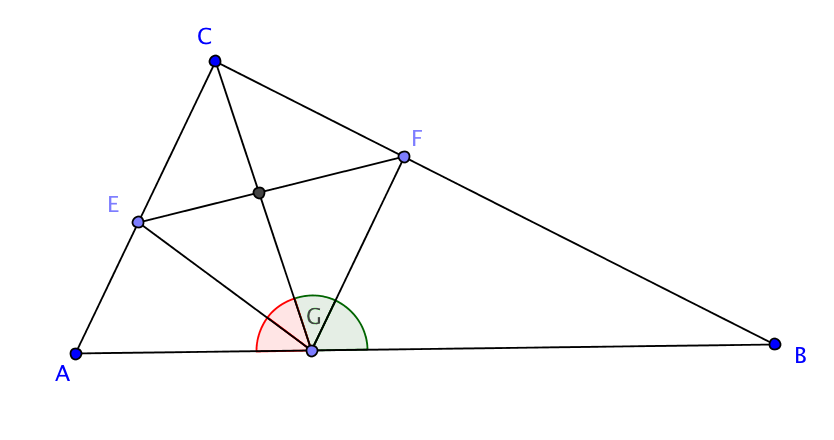# Geometry is not that badIn a triangle $\triangle{ABC}$ we draw a cevian through C that touches AB at G. The angle bisector of $\triangle{BGC}$ touches BC at F and the angle bisector of $\triangle{CGA}$ touches CA at E. If you know $\overline{FG}$ = 33 and $\overline{GE}$ = 56. What is $\overline{EF}$?

×